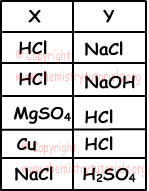## Chemical Reactions Exam1 and Problem Solutions

Chemical Reactions Exam1 and  Problem Solutions

1. Balance following chemical reaction;

C2H5OH + O2 → CO2 + H2O

Solution:

C2H5OH + O2 → CO2 + H2O

We have 2 C atoms in reactants but 1 in products. So, we multiply CO2 with 2 to balance number of C atoms.

C2H5OH + O2 → 2CO2 + H2O

Now we have 6 H atoms in reactants but 2 H atoms in products. To balance them we multiply H2O with 3.

C2H5OH + O2 → 2CO2 + 3H2O

Finally we balance number of O atoms, to balance them we write 3 in front of O2.

C2H5OH + 3O2 → 2CO2 + 3H2O

All atoms are balanced in reactants and products.

2. If given reaction is balanced; find x, y and z numbers in reactants.

CxHy(OH)z + 5O2 → 4CO2 + 5H2O

Solution:

Since reaction is balanced, number of atoms in both sides must be equal.

CxHy(OH)z + 5O2 → 4CO2 + 5H2O

In products we have; 13 O atoms, there must be 13 O atoms in reactants. But we have 10, so "z" must be 3.

In products we have 4 C atoms, to balance it there must be 4 in reactants, so "x"is 4.

C4Hy(OH)3 + 5O2 → 4CO2 + 5H2O

There 10 H atoms in products but 3 in reactants. "y" must be 7 to balance number of H atoms in both sides. Final reaction;

C4H7(OH)3 + 5O2 → 4CO2 + 5H2O

3. Find formula of compound represented with "X" in following balanced reaction.

2I- + 2X + 4H+1 → I2 + 2NO + 2H2O

Solution:

We should check number of atoms in both sides to find formula of X.

There are 2 I atoms in reactants and products, so there is no I atom in X.

There are 4 H atoms in reactants and products, there is no H atom in X.

There are 2N atoms in products but there is no in reactants, so there must be 2 N atoms in reactants.

Since there are 4 O atoms in products there must be 4O atoms in reactants.

X must be "NO2"

2I- + 2NO2 + 4H+1 → I2 + 2NO + 2H2O

Now, we must balance number of charges in both sides.

2I- + 2NO2 + 4H+1 → I2 + 2NO + 2H2O

2.(-1) +2.(x) + 4.(+1) =1.0 + 2.0 + 2.0

-2+2x+4=0

x=-1, so reaction becomes;

2I- + 2NO2-1 + 4H+1 → I2 + 2NO + 2H2O

and X=NO2-1

4. Which ones of the following reactions are acid-base reaction?

I. N2(g) + 3H2(g) → 2NH3(g)

II. Mg(OH)2(s) + 2HCl(l) → MgCl2(s) + 2H2O(l)

III. CH4(g) + 2O2(g) → CO2(g) + 2H2O(l)

Solution:

I. It is synthesis or formation reaction.

II. Mg(OH)2 is base and HCl is acid.Thus, it is acid base reaction; salt and water are formed.

III. This is combustion reaction.

5. Which one of the following couples can be written into the place of X and Y in reaction below;

X + Y → Salt + H2OSolution:

X + Y → Salt + H2O is an acid-base reaction. Thus, X and Y must be acid and base or base and acid.

HCl + NaOH  → NaCl + H2O

X       Y

Related